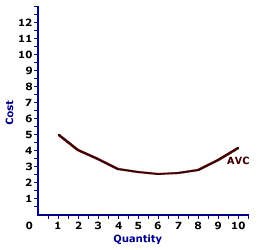Friday  June 2, 2023
 AmosWEB means Economics with a Touch of Whimsy!PERFECT COMPETITION, LONG-RUN PRODUCTION ANALYSIS: In the long run, a perfectly competitive firm adjusts plant size, or the quantity of capital, to maximize long-run profit. In addition, the entry and exit of firms into and out of a perfectly competitive market guarantees that each perfectly competitive firm earns nothing more or less than a normal profit. As a perfectly competitive industry reacts to changes in demand, it traces out positive, negative, or horizontal long-run supply curve due to increasing, decreasing, or constant cost.AVERAGE VARIABLE COST CURVE:

A curve that graphically represents the relation between average variable cost incurred by a firm in the short-run product of a good or service and the quantity produced. This curve is constructed to capture the relation between average variable cost and the level of output, holding other variables, like technology and resource prices, constant. The average variable cost curve is one of three average curves. The other two are average total cost curve and average fixed cost curve. A related curve is the marginal cost curve.
The average variable cost curve is U-shaped. Average variable cost is relatively high at small quantities of output, then as production increases, it declines, reaches a minimum value, then rises. This shape of the average variable cost curve is indirectly attributable to increasing, then decreasing marginal returns (and the law of diminishing marginal returns).

Average Variable Cost CurveThis graph is the average variable cost curve for the short-run production of Wacky Willy Stuffed Amigos (those cute and cuddly armadillos and tarantulas). The quantity of Stuffed Amigos production, measured on the horizontal axis, ranges from 0 to 10 and the average variable cost incurred in the production of Stuffed Amigos, measured on the vertical axis, ranges from a high of \$5 to a low of \$2.50, before rising again.

As noted above, the average variable cost curve is U-shaped. For the first 6 Stuffed Amigos, average variable cost declines from over \$5 to a low of \$2.50. However, for the production of 7 (or more) Stuffed Amigos, average variable cost increases.

The average variable cost curve is most important to the analysis of a firm's decision to shut down production in the short run. If price is greater than average variable cost, then a firm may or may not be receiving an economic profit, but it is better off producing in the short run than shutting down production. Shutting down production entails a loss equal to total fixed cost. However, with price greater than average variable cost, sufficient revenue is generated to pay ALL variable cost and some fixed cost, making the operating loss less than fixed cost.

If price is less than average variable cost, then a firm incurs a loss greater than total fixed cost by producing. Its operating loss includes both fixed cost, plus part of the variable cost not covered by the price. As such, the firm is better off shutting down production and awaiting better times.

 <= AVERAGE VARIABLE COST AXIOM =>Recommended Citation:

AVERAGE VARIABLE COST CURVE, AmosWEB Encyclonomic WEB*pedia, http://www.AmosWEB.com, AmosWEB LLC, 2000-2023. [Accessed: June 2, 2023].

Check Out These Related Terms...

Or For A Little Background...

And For Further Study...
Search Again?BLACK DISMALAPOD[What's This?] Today, you are likely to spend a great deal of time looking for a downtown retail store wanting to buy either rechargeable batteries or a rechargeable battery for your computer. Be on the lookout for slow moving vehicles with darkened windows.Your Complete ScopeThe average bank teller loses about \$250 every year."I know the price of success; dedication, hard work and an unremitting devotion to the things you want to see happen. "-- Frank Lloyd Wright, architectMCPMarginal Cost PricingA PEDestrian's Guide Xtra CreditTell us what you think about AmosWEB. Like what you see? Have suggestions for improvements? Let us know. Click the User Feedback link.| | | | | | | | | | |
| | | |

Thanks for visiting AmosWEB